# CBSE Class 9 Science Gravitation And Fluid Notes Set B

GRAVITATION AND FLUID

INTRODUCTION

Besides developing the three laws of motion, Sir lssac Newton also examined the motion of the heavenly bodes - the planets and the moon. Newton recognized that a force of some kind must be acting on the planets to keep them in nearly circular orbits, otherwise their paths would be straight lines. A falling apple is attracted by the earth by the apple attracts the earth as well (Newton’s third law of motion). Extending this idea, Newton proposed that every body in this universe attracts every other body. This led to the discovery of the famous law of universal gravitation i.e. each object in this universe attracts every other object. Note that gravitational force is attractive. Newton concluded that it was the gravitational force that acted between the sun and each of the planets to keep them in their orbits. In this chapter, we shall discuss the role of gravitational force of the earth of the objects, on or nor the surface of the earth.

(a) Gravitation or Gravitational Force :

It was Newton, who said that every object in this universe attracts every other object with a certain force. The force with which two objects attract each other is called the force of gravitation. The force of gravitation acts even if the two objects are not connected by the any means. If, however, the masses of the objects are small, the force of gravitation between them is small and cannot be detected easily.

The force of attraction between any two particles in the universe is called gravitation or gravitational force.

NEWTON’S LAW OF GRAVITATION

The magnitudes and the direction of the gravitational force between two particles are given by the universal law of gravitation, which was formulated by Newton.

Universal law of gravitation :

The gravitational force of attraction between two particles is directly proportional to the product of the masses of the particles and is inversely proportional to the square of the distance between the particles. The direction of the force is along the line joining the two particles.

Mathematical derivation :

Let A and B be two particles of mass m1 and m2 respectively. Let the distance AB = r. By the law of gravitation, the particle A attracts the particle B with a force F such that,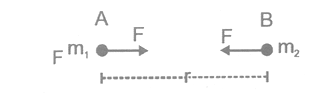F α m1m2            (for a given pair of particles)

and  F α1/r2       (for given separation between the particles)

So   F α m1m r2

or    F=G m1m/r2

Here G is a constant known as the universal constant of gravitation.

(a) Universal Gravitational Constant :

(i) Introduction :

Force of gravitation between two bodies of mass m1 and m2 kept with distance r between their centres, is given by :F=G m1m/r2

where constant of proportionality G is called universal gravitational constant (U.G.C.).

(ii) Definition :

In relation    F=G m1m/r2

If m1 = m2 = 1, r = 1, then F = G Hence, universal gravitational constant may be defined as the force of attraction between two bodies of unit mass each, when kept with their centres a unit distance apart.(b) Important Characteristics of Gravitational Force :

(i) Gravitational force between two bodies form an action and reaction pair i.e., the forces are equal in magnitude but opposite in direction.

(ii) Gravitational force is a central force i.e., it acts along the line joining the centres of the two interacting bodies.

(iii) Gravitational force between two bodies is independent of the nature of the intervening medium.

(iv) Gravitational force between two bodies does not depend upon the presence of other bodies.

(v) Gravitational force is negligible in case of light bodies but becomes appreciable in case of massive bodies like starts and planets.

(vi) Gravitational force is a long range force i.e., gravitational force between two bodies is effective even if their distance of separation is very large. For example, gravitational force between the sun and the earth is of the order of 1022 N, although distance between them is 1.5 × 108 km.

(vii) Gravitational force is a conservative force.

(c) Experimental Support for the Law of Gravitation :

(i) All the planets including the earth, rotate around the sun due to gravitational force between the sun and the planet.

(ii) Tides are formed in oceans due to gravitational force between the moon and the earth.

(iii) It is the gravitational force between the planet and its satellite which makes the satellite to move around the planet.

(iv) The atmosphere of the earth is due to the gravitational force of the earth.

NEWTON’S THRID LAW OF MOTION AND GRAVITATION

Newton’s third law of motion says that : If an object exerts a force on another object, then the second object exerts an equal and opposite force on the first object. The Newton’s third law of motion also holds good for the  force on the earth in the opposite direction. Thus, even a falling object attracts the earth towards itself. When an object, say a stone, is dropped from a height, it gets accelerated and falls towards the earth and we say that the stone comes down due to the gravitational force of attraction exerted by the earth. Now, the stone also exerts and equal and opposite force on the earth, then why don’t we see the earth rising up towards the stone.

From Newton’s second law of motion, we know that :

Force = Mass × Acceleration

So,   Acceleration = Force /  Mass

or   a= F/M

It is clear from this formula that the acceleration produced in a body is inversely proportional to the mass of the body. Now, the mass of a stone is very small, due to which the gravitational force produces a large acceleration in it. Due to large acceleration of stone, we can seen the stone falling towards the earth. The mass of earth it, however, very-very large. Due to the very large mass of the earth, the same gravitational force produces very-very small acceleration in the earth. Actually, the acceleration produced in the earth is so small that it cannot be observed. And hence we do not see the earth rising up towards the stone.

ILLUSTRATIONS

1. Two persons having mass 50kg each, are standing such that the centre of gravity are 1m apart. Calculate the force of gravitation and also calculate the force of gravity on each.

Sol. Given : m1 = m2 = 50kg.F’ is much greater than F so the persons will not move towards each other but each of them moves towards the earth.

ESTI MATION OF GRAVITATIONAL FORCE BETWEEN DIFFERENT OBJECTS

(a) Between Sun and Earth :

Mass of earth, m1 = 6 × 1024 kg

Mass of the moon, m2 = 7.4 × 1030 kg

Distance between the sun and the earth, r = 1.5 × 1011m

Gravitation force between the sun and the earth,The gravitational force between the sun and the earth is very large (i.e. 3.6 × 1022 N). This force keeps the earth bound to the sun.

(b) Between Moon and Earth :

Mass of the earth, m1 = 6 × 1024 kg

Mass of the moon, m2 = 7.4 × 1022 kg

Distance between the earth and the moon, r = 3.8 × 108 m

Gravitational force between the earth and the moon,

F = Gm1m2/ r2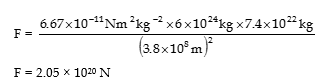This large gravitational force keeps the moon to move around the earth. This large gravitational force is also responsible fort the ocean tides.

1. Two bodies A and B having mass m and 2m respectively are kept at a distance d apart. Where should a small particle be placed so that the net gravitational force on it due to the bodies A and B is zero ?

Sol.    it is clear that the particle must be placed on the line AB, suppose it is at a distance x from A.

Let its mass is m’.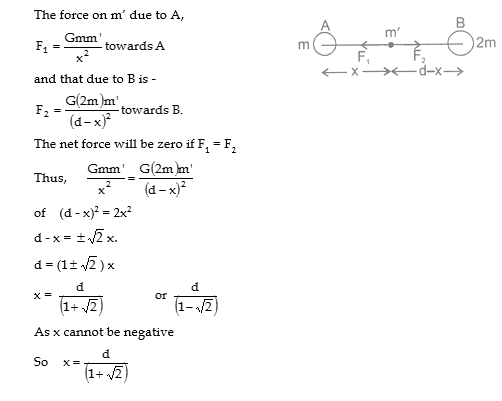FORCE OF GRAVITATION OF THE EARTH (GRAVITY)

Gravitation and gravity :

Attraction between two bodies having mass of same order, is called gravitation and the force is called gravitational force. Forces involved are very small and the attracting bodies do not move towards each other.

Attraction between a planet (earth) or its satellite and a body, having masses of widely different order is called gravity and the force is called force of gravity. Forces involved  are large and body moves towards the planet.

Thus, gravity becomes a special case of gravitation in which small bodies move towards huge planets. Then force of gravity

F= GmM / r2

BODIES FALLING NER THE SURFACE OF THE EARTH

(a) Galileo’s Observations on Falling Bodies :

The speed of falling body increases as it comes down. This means that the body accelerates, when i t falls freely. Suppose we drop a coin and a feather from the same height simultaneously. Which will reach the ground first ? The answer is obvious, the coin will reach earlier than lighter feather or we can say that the heavier objects comes down more faster than lighter ones but such a generalization is not correct. If we take two slid balls of different masses, say, one of 1 kg and the other of 2kg, and drop them from the same height, we will find that they reach the ground almost simultaneously.

It is said that Galileo dropped two stones of different masses from the Leaning Tower of Pisa (in Italy) and founded that they reached the ground simultaneously. Galileo argued that the air resist on object traveling through it. If the material is dense and its surface area is small, the resistance due to air is quite small compared to the force of gravity. Thus one can neglect the effect of air resistance while studying falling stones, metallic blocks, coins etc. But the effect of air resistance is very important for small pieces of paper, feather, leaves etc. each of which ahs a large surface area and low density. When a coin and a feather fall through air, air offers greater resistance to the motion of the feather and les resistance to the motion of the coin, According to Galileo’s argument, if air is totally removed, the coin and the feather will fall simultaneously.

Newton was born in the year Galileo died. Galileo died not have access to the equations for gravitational attraction and the acceleration resulting from a force. Still, he correctly predicted something from his observations that was contrary to everyday experience.

Galileo’s prediction was tested by the British scientist Robert Boyle. He kept a coin and a feather in a long glass tube and evacuated the air from inside the tube by using a vacuum pump. When the tube was inverted, the coin and the feather fell together.

(b) Acceleration due to Gravity :

if we drop a ball from a height, its speed increases as time passes. If we throw a ball upwards, its speed decreases till it reached the highest point. If we throw the ball at an angle to the vertical, its direction of motion changes. In all these cases, the velocity of the ball changes, i.e., the all is accelerated, whenever an object moves near the surface of the earth with no other object pushing or pulling it, it is accelerated. This acceleration is caused due to the force of gravity and is called the acceleration due to gravity. Consider an object of mass m moving freely near the earth’s surface. Neglecting air resistance, the only force on it, is due to gravity. The force has magnitude :

F= GM6m / R2 e ........(i)

where M = mass of the earth, m = mass of the object, and Re = radius of the earth.

As the earth’s radius Rs (6400 km) is large as compared to distance of the object from the earth’s surface. We use Re in Equation (i) to denote the distance of the object from the centre of the earth. As the force given by equation (i), is the resultant force on the object, its acceleration is

a= F / M = GMe / R2e

Note that this acceleration does not depends on the mass of the object. Thus we have the following :

if gravity is the only acting force (meaning that air resistance is neglected), all objects move with the same acceleration near the earth’s surface. This acceleration is called the acceleration due to gravity, whose magnitude ‘g’ is given byThe direction of this acceleration is towards the centre of the earth, i.e., in the vertically downward direction. The acceleration has the same value, both in magnitude (9.8 m/s2) and direction (towards centre of earth), whether the particle falls, moves up or moves at some angle with the vertical. In all these cases, we say that the particle moves freely under gravity.

(c) Value of ‘g’ on the Surface of the Moon :

g= GM / Rwhere M is the mass of a heavenly body like earth and R is its radius. As all heavenly bodies (like planets, the sun and the moon) are of different masses and different radii, so the value of g is different on different heavenly bodies.Thus acceleration due to gravity on the surface of moon is  times the acceleration due to gravity on the surface of the earth.

MASS OF EARTH AND MEAN DENSITY OF EARTH

(a) Mass of the Earth :

The mass of the earth can be calculated by using Newton’s law of gravitation. Consider a body of mass m lying on the surface of the earth, then

force of gravity acting on the body is given by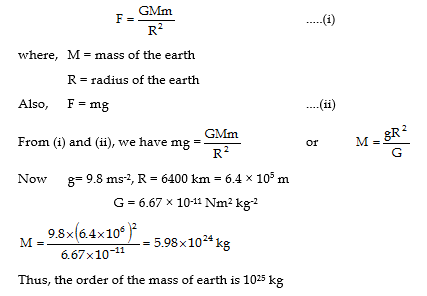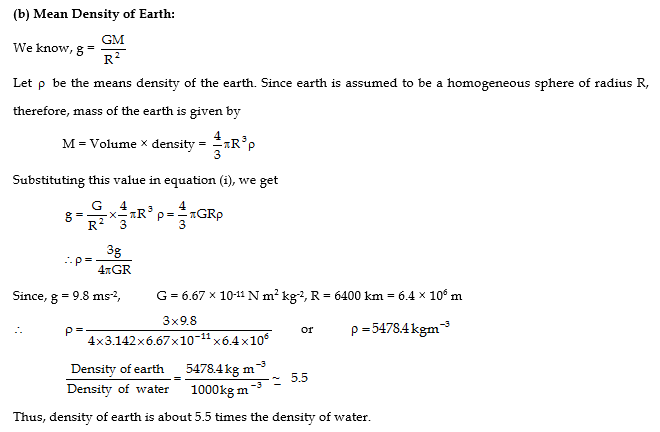Since the freely falling bodies fall with uniformly accelerated motion, the three equations of motioning derived earlier for bodies under uniform acceleration can be applied to the motion of freely falling bodies. For freely falling bodies, the acceleration due to gravity is ‘g’ so we replace the acceleration ‘a’ of the equations by ‘g’ and since the vertical distance of the freely falling bodies is known as height ’h’, we replace the distance ‘s’ in our equations by the height ‘h’. This gives us the following modified equations for the motion of freely falling bodies.

We shall use these modified equations to solve numerical problems. Before we do that, we should remember the following important points for the motion of freely falling bodies.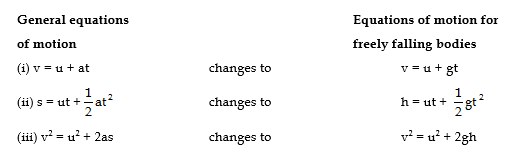(i)  When a body is dropped freely from a height, its initial velocity ‘u’ becomes zero.

(ii) When a body is thrown vertically upwards, its final velocity ‘v’ becomes zero.

(iii) The time taken by a body to rise to the highest point is equal to the time it takes to fall from the same height.

(iv) The distance traveled by a freely falling body is directly proportional to the square of time of fall.

(a) Sign Conventions :

(i) g is taken as positive when it is acting in the same direction as that of motion and g is taken as negative when it is opposing the motion.

(ii) Distance measured upward from the point of projection is taken as positive, while distance measured downward from the point of projection is taken as negative.

(iii) Velocity measured away from the surface of earth (i.e. in upward direction) is taken as positive, while velocity measured towards the surface of the earth is taken as negative.

MASS AND WEIGHT

(a) Mass :

Definition : Quantity of matter possessed by a body, is called the mass of the body. It is represented by the symbol m. It is a clear quantity.

Nature : A body with more mass, needs a greater effort (force) to move it from rest or stopping it from motion. The body exhibits inertia. Thus, mass offers inertia. This mass is called inertial mass (m1).

A body never has a zero  mass.

Measurement of mass :

Mass of a body is measured by a beam balance by comparing the mass with bodies of known mass. At one place, bodies of same mass have same pull of gravity on them.

A beam balance works on the principle of moments (Bodies of equal masses, having equal weights, have equal and opposite moments about fulcrum of the balance, when suspended at equal distances from the fulcrum, and made the beam horizontal).

(b) Weight:

Definition :The force with which a body is attracted towards the centre of the earth, is called the weight of the body. It is represented by the symbol W.

It is a vector quantity having direction towards the centre of the earth.

Expression for weight :

If mass of a body = m

Acceleration due to gravity of the earth = g

Then from relation ,

Force = Mass × Acceleration    i.e., W = mg

This is the required expression.

Nature :As W = mg, the weight of a body will vary from place to place due to variation in value of g. A body has zero weight at the centre of the earth (where g = 0).

Measurement of weight :

Weight of a body is measured by a spring balance.

(C) Difference between Mass and Weight: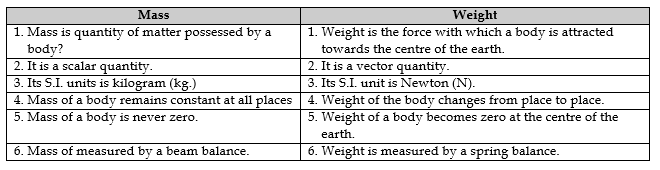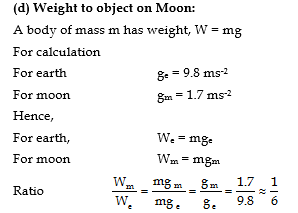i.e. Weight ton moon = 1/6 th weight on earth.

kg.wt. is a unit of force:

From relation,     W = mg

If m = 1kg             W = 9.8 N

Hence a 1 kg body has weight of 9.8 N

It means that 9.8 N becomes equal to a force of 1 kilogram weight (kg. wt.)

(e) Variation in the weight of a body :

Weight of the body is given by,

W = mg

So the weight of a body depends upon (i) the mass of the body and (ii) value of acceleration due to gravity (g)at a place.

The mass of a body remains the same throughout the universe, but as the value of ‘g’ is different at different places. Hence, he weight of a body is different at different planes.

(i) The value of ‘g’ is more at poles and less at the equator. Therefore, weight of a body is more at the poles and less at the equator. In other words, a body weighs more at the poles and less at the equator.

(ii) The value of ‘g’ on the surfaces of different planet of the solar system is different, therefore, the weight of a body is different on different planet’s

(iii) The value of ‘g’ decreases with height from the surface of the earth. Therefore, the weight of a body also decreases with height from the surface of the earth. That is why, the weight of a man is less on the peak of Mount Everest that the weight of the man at Delhi.

(iv) The value of ‘g’ decreases with depth from the surface of the earth. Therefore, the weight of a body decreases with depth from the surface of the earth.

(v) The value of ‘g’ at the centre of the earth is zero hence weight (=mg) of the body is zero at the centre of the earth.

DIFFERENCE BETWEEN ‘g’ AND ‘G’WEIGHTLESNESS

(a) Introduction:

When a man stands on weighing machine at rest, his weight compressed its spring downwards. Due to upward reaction, the pointer of the machine moves over the scale and the machine records the weight of the man.

But when the same machine starts falling down freely, there is no reaction and the pointer stays at zero recording a zero weight.

The man falling freely under the action of gravity has become weightless.

Definition : Weightlessness may be defined as the state in which a body its weight due to free fall.

(d) Demonstration : Let a stone piece be suspended from a spring balance suspended by a hand finger. The balance shows the actual weight of the stone.

When the balance is released from h and finger, the balance falls freely with the hanging stone piece. The balance shows a zero reading. This proves that the freely falling stone is weightless.

(i) The spring balance shows the weight of the stone.

(ii) Freely falling spring balance with the stone showing a zero reading.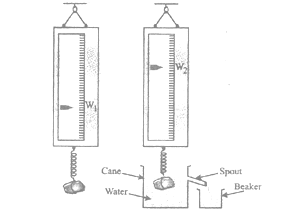(c) Weightlessness of an Astronaut in a Satellite (Space Ship):

A satellite is a freely falling body orbiting round the earth. It tries to reach the earth but its path being parallel to earth’s surface. It does not reach the earth. Hence the satellite and all the bodies inside it become weightless.

It is due to this situation of weightlessness of astronauts that they are shown floating in spaceship in films   on television.

FLUID

Fluid is a substance that flows under the action of an applied force and does not have a shape of its own. For example, liquids and gases. They take the shape of container in which they are stored.

The study of fluids at rest is known as hydrostatic or fluid static’s. The study of fluids in motion is termed as hydrodynamics. In this chapter, we will discuss the study of liquids only.

PRESSURE IN A FLUID

In case of solids, the force can be applied in any direction with respect to the surface, but in liquids, the force must be applied at right angles to the liquids surface. This is because fluids (liquids and gases) at rest cannot sustain a tangential force. Therefore, we state the pressure acting on the fluid instead of force.

The pressure (P) is defined at the magnitude of the normal force acting on a unit surface area of the fluid. It a constant force of magnitude F acts normally on a surface area A, then pressure acting on the surface is given by. The pressure is a scalar quantity. This is because hydrostatic pressure is transmitted equally in all directions when force is applied, which shows that a definite direction is not associated with pressure.

THRUST

The total force exerted by a liquid on any surface in contact with it is called thrust of the liquid.

Thrust = Pressure × Area of surface

UNITS OF PRESSURE

In C.G.S. system, unit of pressure is dyne/cm2. S.I. unit of pressure is Nm-2 or Pascal (PA). The unit of pressure, Pascal (Pa) has been named in the honour of great French scientist and philosopher Blasie Pascal.

Another unit of pressure is atmosphere (atm).

1 atm = 1.013 × 105 Nm-2 (or Pa)

1 atm. or one atmosphere is the pressure exerted by our atmosphere on earth surface due to the weight of atmosphere.

(a) Pressure is a Scalar Quantity :

Scalar quantities are those which do not have any direction. The physical quantities which have both magnitude and direction are called vector quantities. Pressure is a scalar quantity because at one level inside the liquid, the pressure is exerted equally in all direction, which shows that a direction is not associated with hydrostatics pressure or pressure due to a static fluid.

(b) Pressure Exerted by a Liquid Column :

Consider a liquid of density  contained in a cylindrical vessel of cross sectional area a. Let h be the height of liquid column and g be the acceleration due to gravity. The weight of liquid will exert a downward thrust on the bottom surface of the vessel. Therefore, pressure due to liquid acts on that surface.

Weight of liquid inside the vessel = volume × density of liquid × acceleration due to gravity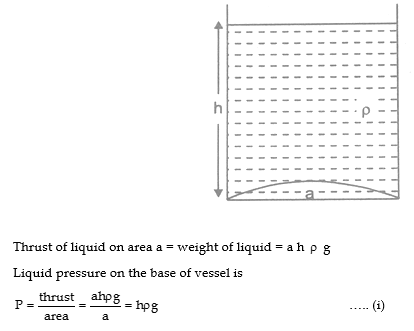NOTE :

(i) The liquid at rest exerts equal pressure in all direction s at a point inside the liquid.

(ii) The liquid at rest exerts equal pressure at all those points which are in one level inside the liquid.

(iii) Liquid pressure is independent of shape of the liquid surface, but depends upon the height of liquid column.

(iv) Total pressure at a depth h below the liquid surface = P0 +  where P0 = atmospheric pressure.

(v) Pressure is a scalar quantity.

(vi) Mean pressure on the walls of a beaker containing liquid upto height is (=/2 ), where  is the density of liquid.

(vii) Thrust exerted by liquid on the walls of the vessel in contact with liquid is normal to the surface of vessel.

(C) Some Facts Involving Thrust and Pressure :

(i) Nails have a flat top but pointed end:

A small pressure applied on the flat to through falling hammer becomes a large thrust. The same thrust acts on the wooden board through the pointed end of the nail.  It result in a large pressure. The nail can easily be fixed in the wooden board.

(ii) Sewing needle have pointed tips :

A small force of fingers makes the needle pierce into the cloth easily and sewing becomes quicker.

(iii) Cutting items (knives and blades) have sharp edge. Cutting becomes easier.

(d) Reducing Pressure :

(i) Vehicle brakes have flat surface :

This reduces pressure on the vehicle tyres and avoid their tearing.

Broad sole shoes make walking easier on a soft land.

(iii) Wide steel belt on army tank :

Wide steel belt over the wheels of an army taken, makes its movement easier over marshy land.

(vi) Tractor tyres are broad : Tractors do not sink in the soft land of the field while operating them.

(v) Camel foot are broad and soft : They walk swiftly on sand.

(vi) Hanging bags have wide straps : They reduce pressure on the shoulders.

BUOYANCY AND FORCE OF BUOYANCY (BUOANT FORCE)

Introduction : When a body is immersed in a fluid (liquid or gas), it displaced the fluid whose volume is equal to the volume of the body immersed in the fluid. This displaced fluid exerts an upward force on the body.

Definition : This tendency of the displaced fluid (exerting an upward force) is called buoyancy. The upward applied force, is called the force of buoyancy or up thrust. It is equal to the weight of the fluid displaced by the body.

Examples :

(i) When a bucket is pulled out of a well, it is felt lighter so long as it remains immersed in water, inside the well. It acquires its actual weight when out of water.

(ii) Ladies carrying water in a pitcher from a village pond, enter the pond, fill the pitcher and lift it on to their shoulder keeping it immersed in water.

Factors on which up thrust or buoyant force depends :

Let us perform the following two activates :

(i) Take two wooden blocks of different  sizes. Push the small block inside the water in a tub and release it. You will find that the wooden block rises up and come to the surface of water. It rises up because upthrust or buoyant force acting on it is more than its weight.

Now push the large wooden block inside the water and release it. You will find that the large block rises up faster than the small block. It means, the upthurst or buoyant force acting on the large block is more than on the small block.

(ii) Now add some salt in water so that the density of solution (water + salt) increases. Push a wooden block inside the solution and release it. You will find that the block rises up faster in a solution than in pure water. It means, the upthrust or buoyant force acting on a body is more in a liquid having more density than in a liquid having less density.

Conclusion :

From the above mentioned actives, we conclude that upthrust or buoyant force depends on :

(i) The size or volume of the body immersed in a liquid.

(ii) The density of the liquid in which the body is immersed.

Buoyancy:

The tendency of an object to float in a liquid or the power of liquid to make an object float is called  buoyancy.

Remember these points:

(i) An object whose weight (i.e., downward gravitational force) is greater than the upthrust of the liquid (say water) on the object, sinks in the liquid. This is possible if density of object is more than the density of liquid.

(ii) An object whose weight (i.e. downward gravitational force) is less than the upthurst of the liquid on the object, floats on the liquid. This is possible if density of object is less than the density of liquid.

ARCHEMED PRINCIPLE

A Greek scientist Archimedes conducted many experiments and concluded that when a body or an object is immersed partially or completely in a liquid or gas (i.e. fluid), it experiences an up thrust or buoyant force. The up thrust or buoyant force is equal to the weight of the fluid displaced by the body. It is known as Archimedes principle.

Statement of Archimedes principle:

When a body is immersed partially or completely in a fluid (liquid or gas), it experiences an up thrust or buoyant force which is equal to the weight of the fluid displaced by the body.

(a) Proof of Archimedes Principe:

Consider a cylindrical body of cross-sectional area ‘a’ submerged in a liquid of density. Let the upper face of the body is at a depth hbelow the surface of the liquid and the lower face is at a depth h2 below the surface of the liquid. The pressure exerted by the liquid on the upper surface of the body is given by p1 = h1ρgWhich implies that products of the volume of the body, the density of the liquid and the acceleration due to gravity gives the weight of the liquid displaced.

Thus, when a body is submerged in a liquid, it experiences an upward thrust equal to the weight of the liquid displaced by the body.

(b) Verification of Archimedes Principle:

To verify the Archimedes Principles we take following steps:(i) Take a small piece of stone and suspend it with a spring balance. Let the weight of the stone indicated by the spring balance be W1.

(ii) Now take an empty beaker and measure its weight by suspending it with the spring balance with the help of a thread of negligible mass. Let the weight of the empty beaker be W2.

(iii) Take a Cane having a side tube known as spout. Fill Cane with water upto the level of spout.

(vi) Lower the stone suspended with a spring balance inside the water. The stone displaces the water which comes out of the Cane through the spout. The water coming out of the Cane is collected in the beaker. When the water stops coming out of the spout, note the reading of the spring balance. This reading shows the weight of the stone inside the water. Let the weight of the stone inside the water be W3. It is seen that W3 is less than W1.

(v) Now measure the weight of the beaker along with the water collected in it. Let this weight be W4.

(vi) Now find (W1 - W3). This difference in weight is equal to the loss of weight of the stone immersed in water (i.e. up thrust or buoyant force).

(vii) Also find (W4 - W2). This difference in weight is equal to the weight of the water displaced by the stone.

(viii) It is found that (W1 - W3) = (W4 - W2). That is up thrust or buoyant force is equal to the weight of the water displaced. Thus, Archimedes principle is verified.

DENSITY

The ratio of mass and volume of the body is known as the density of the material of the body.

Density =mass / volume

ρ = M / V

IF V = 1 m3, then, ρ = M

or the mass per unit volume is known as the density of the material of the object.

Unit of density:

ρ= M / V →g / cm3 (in  C.G.S)

ρ  Kg/m3 (in S.I. system)

RELATIVE DENSITY OR SPECIFIC GRAVITY)

The ratio of density of the substance to the density of water at 40C is known as the relative density of the substance.

Relative density of substance = density of substance / density of water at 40C

It is pure number having no unit.

ILLUSTRATION

1. The relative density of silver is 10.5. The density of water is 103kg/m3. What is the density of silver in S.I. unit ?

Sol.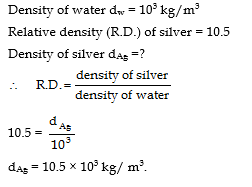USES OF ARCHIMEDES PRINCIPLE

Relative density for solids and liquids can also be determined with the help of Archimedes principle.

(i) For solids :

By definition, we have(ii) For liquids :

To measure relative density of a liquid, choose a body which can be fully immersed in water as well as in the given liquid. The body is weighed first in air, then fully immersed in water and then fully immersed in that particular liquid.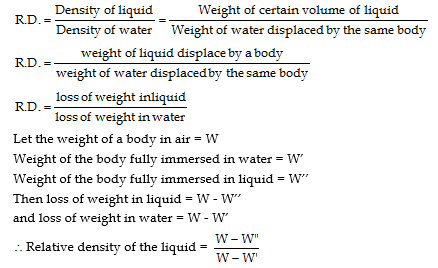(iii) Archimedes principle is used to design:

(A) the ships and submarines.

(B) the hydrometers to find the densities of liquids.

(C) the lactometers to test the purity of milk.

Tale of densities and relative densities of some substances :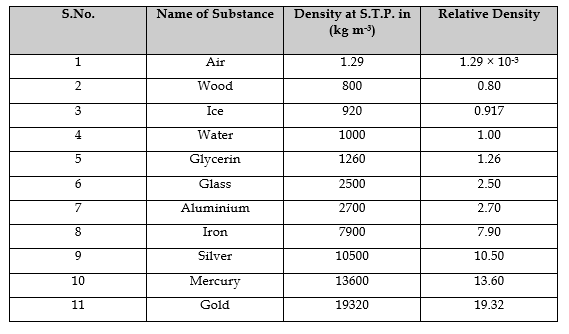PHYSICAL MEANING OF RELATIVE DENSITY

Relative density of a substance is a number of times the given substance is heavier than the equal volume of water. When we say that the relative density of silver = 10.5, it means, silver is 10.5 times heavier than equal volume of water.

LAW OF FLOATATION

Law of floatation is an extension of Archimedes principles.

When a body is immersed partially or wholly in a fluid, then the various forces acting on the body are (i) upward thrust (B) acting at the centre of buoyancy and whose magnitude is equal to the weight of the liquid displaced and (ii) the weight of the body (W) which acts vertically downwards through its centre of gravity.(i) When W > B, the body will sink in the liquid.

(ii) When W = B, then the body will remain in equilibrium inside the liquid.

(iii) When W < B, then the body will come upto the surface of the liquid in such a way that the weight of the liquid displaced due to it balance the weight of body immersed inside the liquid.

Thus law of floatation is defined as follows :

Definition :A body floats is a liquid if weight of the liquid displaced by the immersed portion of the body is equal to the weight of the body.

(a) Relation between Density of Solid and Liquid :

Let  be the density of the solid whose volume if V1. Let ρ2  be the density of the liquid and the volume of the portion of the solid immersed in the liquid be V2.

Now, weight of the floating solid = weight of the liquid displaced.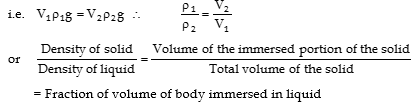(b) Equilibrium of Floating Bodies

From law of floatation, we know that a body will float in a liquid when its weight W is equal to the weight w of the liquid displaced by the immersed part of the body. But this does to necessarily indicate that the body will be in equilibrium. A body will be in equilibrium only if the resultant of all the forces and couples acting on the body is zero. Thus, a floating body can be in equilibrium if no couple acts on it. It will be so if the line of action of W and w is along the same vertical straight line. Thus, there will be equilibrium of floating bodies if the following conditions are fulfilled:

(i) A body can float if the weight of the liquid displaced by the immersed part of body must be equal to the weight of the body.

(ii) A body can be in equilibrium in the centre of gravity of the body and centre of buoyancy must be along the same vertical line.

(iii) The body will be in stable equilibrium if centre of gravity lies vertically above the centre of buoyancy.

NOTE : When an ice block is floating in water in a vessel, then the level of water in the vessel will not change when the whole ice melts into water.

When an ice block is floating in a liquid in a vessel and ice completely melts, then the following cases may arise for the level of liquid in the vessel.

(i) If density of liquid is grater than that of water i.e., the level of liquid plus water will rise.

(ii) If density of liquid is less than the density of water i.e.,   the level of liquid plus water will decrease

(iii) If density of liquid is equal to the density of water i.e., the level of liquid plus water will remain unchanged.

## Tags:

Click for more Science Study Material

## Latest NCERT & CBSE News

Read the latest news and announcements from NCERT and CBSE below. Important updates relating to your studies which will help you to keep yourself updated with latest happenings in school level education. Keep yourself updated with all latest news and also read articles from teachers which will help you to improve your studies, increase motivation level and promote faster learning

### Board Exams Class 10 and 12 Notification by CBSE

Because of the prevailing condition of spread of COVID-19 pandemic in the country, special efforts are required for conducting examinations in a safe manner. As a number of students have shifted from the district of their school to some other district in the country,...

### CBSE Reduced Syllabus Class 9 and 10

The prevailing health emergency in the country and at different parts of the world as well as the efforts to contain the spread of Covid-19 pandemic has resulted in loss of class room teaching due to closure of schools. Therefore the CBSE Board has decided to revise...

### CBSE Decision on Board Exam 2020

The Class 10 and 12 exams are cancelled, and results for both 10th and 12th would be declared by July 15 based on internal exams. CBSE Class 12 students would have the option to appear for the exams at a later date. CBSE has a scheme in which marks scored in past 3...

### CBSE advisory on student marks

CBSE has issued a public advisory for all students to be careful from unscrupulous persons impersonating themselves as officers/officials of CBSE. These people have been contacting parents and have been telling them that they have access to student marks data for Board...

### Board Cancelled Official CBSE Statement

Keeping in view the requests received from various State Governments and the changed circumstances as on date, following has been decided- 1. Examinations for classes X and XII which were scheduled from 1st July to 15th, 2020 stand cancelled. 2. Assessment of the...

### Board Exams helpline by CBSE

According to the recent announcement of CBSE, the pending Class 10 and 12 Board exams are going to be held from 01 July to 15 July 2020 in India. Along with this, it has also been decided to resume the free of cost tele-counseling service for students from 9.30 am to 5...

×